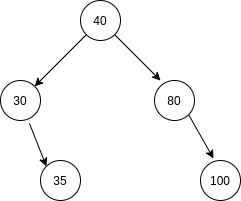New update is available. Click here to update.

# Verify Preorder Sequence in Binary Search Tree

Last Updated: 25 Mar, 2021
Difficulty: Moderate

## PROBLEM STATEMENT

#### You should return true if ‘ARR’ can represent Preorder Traversal of a Binary Search Tree, otherwise return false.##### Example:
``````Consider ‘ARR’ = [40, 30, 35, 80, 100]. You can see that it is the preorder traversal of the Binary Search Tree shown above.

Thus, we should return true in this case.
``````
##### Input format:
``````The first line of input contains an integer ‘T’ denoting the number of test cases. then ‘T’ test cases follow.

The first line of each test case consists of a single integer ‘N’ representing the size of the list/array ‘ARR’.

The second line of each test case consists of ‘N’ single space-separated integers representing list/ array ‘ARR’.
``````
##### Output format :
``````For each test case, print a single line containing a string ‘True’ if  ‘ARR’ can represent Preorder Traversal of a Binary Search Tree, otherwise print ‘False’.

The output of each test case will be printed in a separate line.
``````

#### Note:

``````You do not need to print anything, it has already been taken care of. Just implement the given function.
``````
##### Constraints:
``````1 <= T <= 50
1 <= N <= 10 ^ 4
1 <= ARR[i] <= 10 ^ 9

Where ‘ARR[i]’ is the element of the given array ‘ARR’ at index ‘i’.

Time limit: 1 sec.
``````## Approach 1

For each ‘i’, we can track the closest node for which this ‘i’th node is on the right subtree using stack. After this, we just need to check whether the value of ‘i’th node i.e ARR[i] is greater or smaller than this root value.

Algorithm

• Create an empty stack.
• Initialize an integer variable ‘ROOT’:= 0.
• Run a loop where ‘i’ ranges from 0 to ‘N’ - 1 and for each ‘i’ do the following -:
• If ‘ARR[i]’ < ‘ROOT’, then return False.
• Keep removing elements from the stack while ARR[i] is greater than the stack top and the stack is not empty. Assign the last removed element to ‘ROOT’. If no element is removed, then ‘ROOT’ will not change.
• Push ‘ARR[i]’ in the stack.
• Return True.